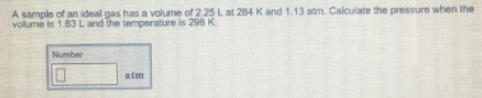# Problem: A sample of an ideal gas has a volume or 2.25 L at 284 K and 1.13 atm. Calculate the pressure when the volume is 1.83 L and the temperature is 298 K.

###### FREE Expert Solution

Combined gas law states that:

88% (70 ratings)###### Problem Details

A sample of an ideal gas has a volume or 2.25 L at 284 K and 1.13 atm. Calculate the pressure when the volume is 1.83 L and the temperature is 298 K.